## "Bottom Line: 6th Grade Math offers and easy to use review and practice app covering all of the basics of math for the grade level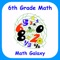# 6th Grade Math - Math Galaxy

by Math Galaxy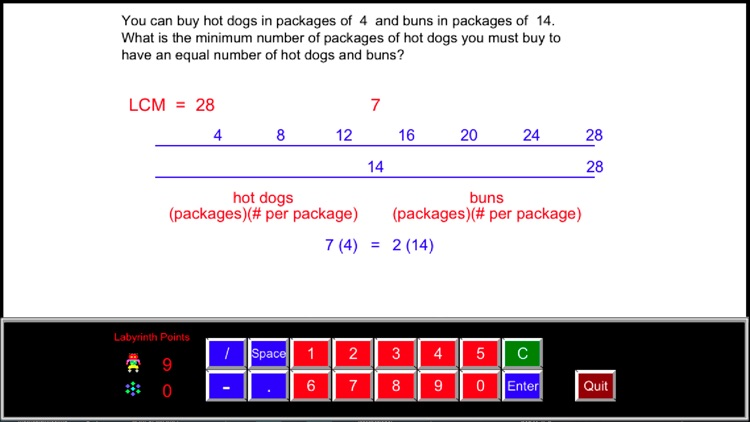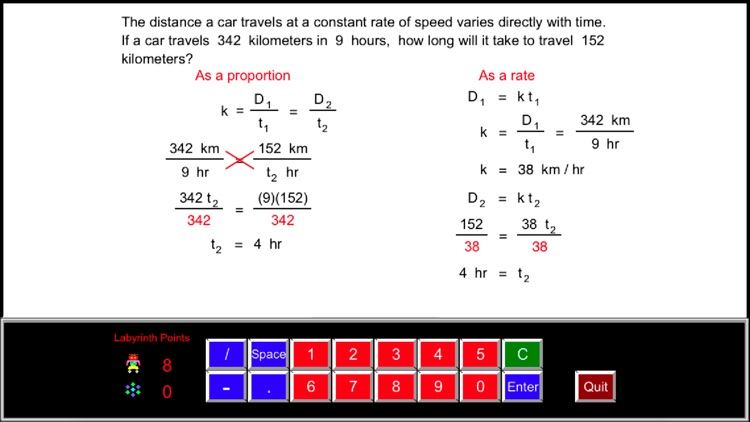"Bottom Line: 6th Grade Math offers and easy to use review and practice app covering all of the basics of math for the grade level. This basic, no-frills app gives kids a great resource to help with comprehension of required math skills for 6th grade."### App Details

Version
3.1
Rating
NA
Size
286Mb
Genre
Education Casual
Last updated
June 21, 2019
Release date
December 10, 2014

### App Screenshots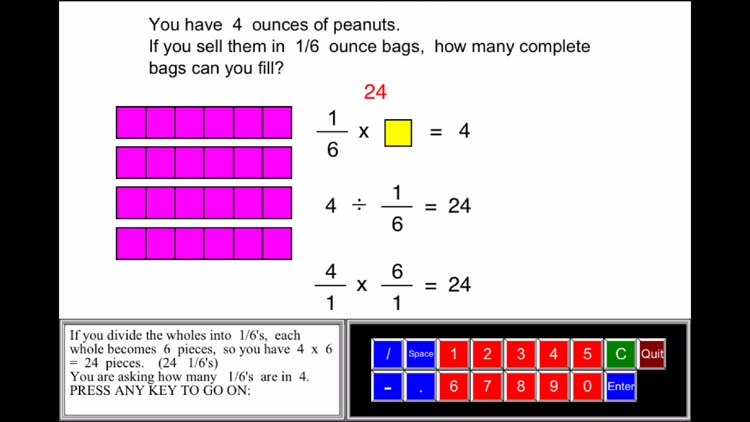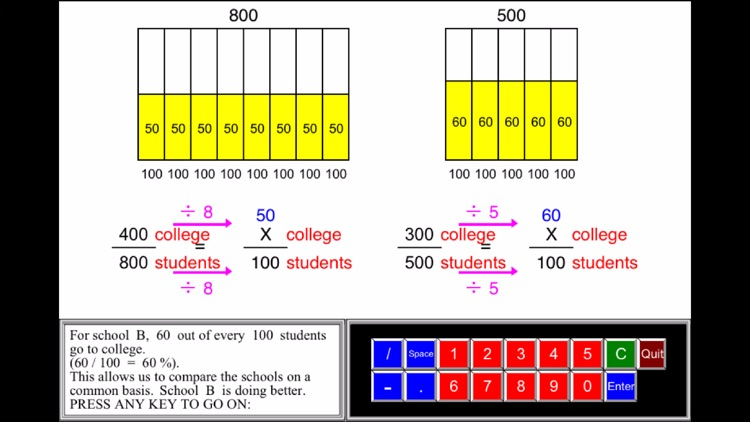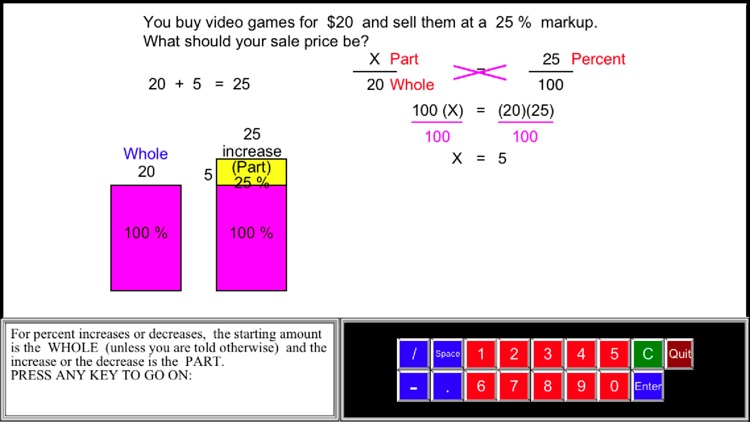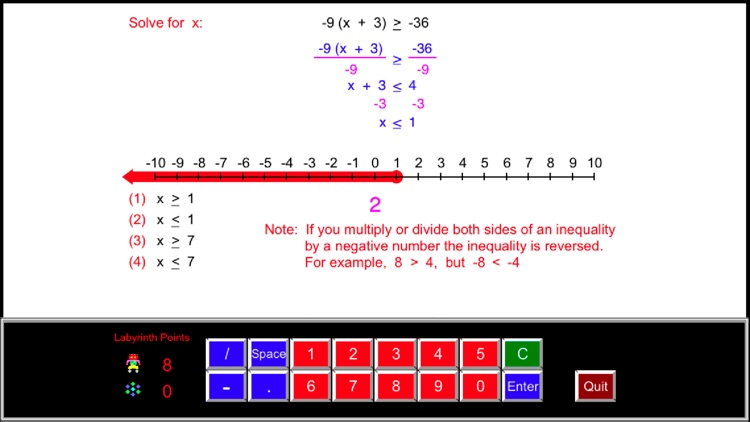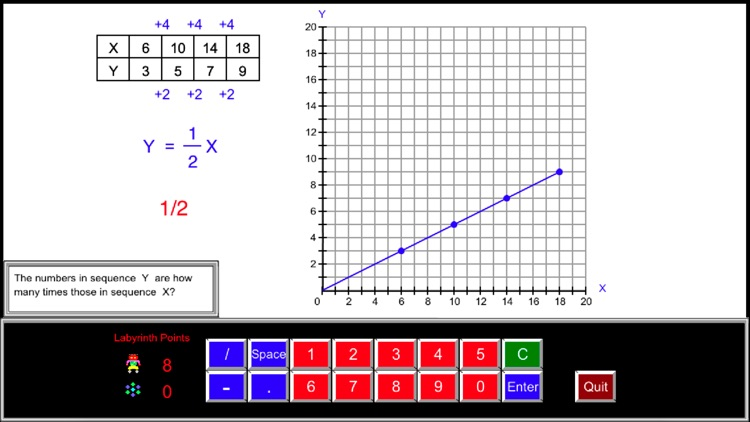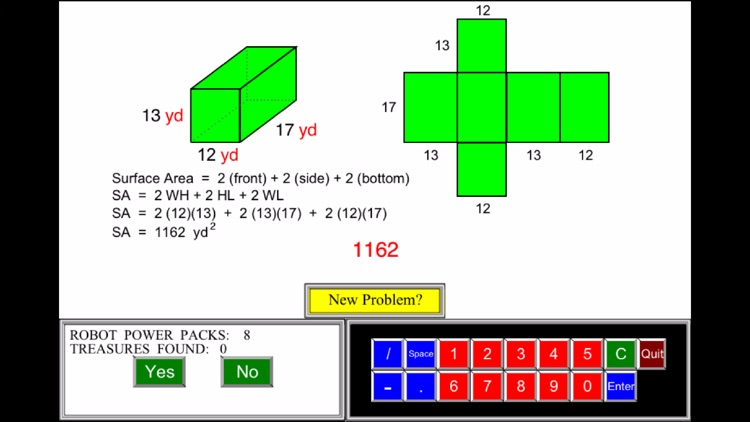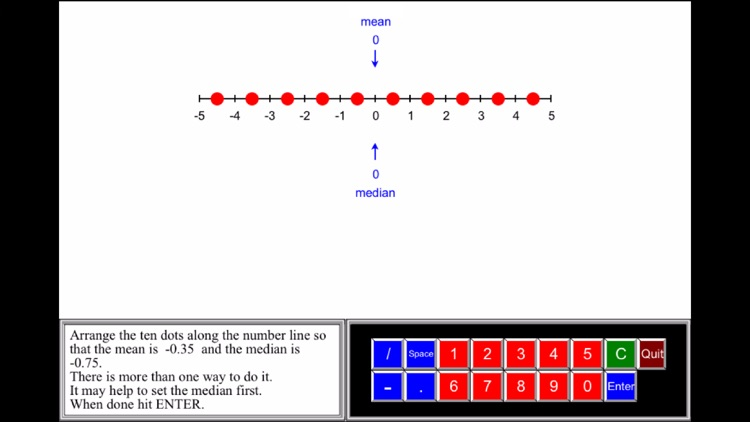### App Store Description

"Bottom Line: 6th Grade Math offers and easy to use review and practice app covering all of the basics of math for the grade level. This basic, no-frills app gives kids a great resource to help with comprehension of required math skills for 6th grade."
See the rest of the review at:

Math Galaxy’s Sixth Grade Math covers all of the Common Core math standards for sixth grade as listed below.
The concepts are presented in bite-size pieces with an unlimited supply of practice problems, with numbers chosen randomly.
Illustrations are used throughout to reinforce the concepts.
By solving problems students earn robots to use in a maze game.
Certificates and summaries of scores can be emailed to be printed.
A stylus is recommended with iPhones.

RATIOS and PROPORTIONAL RELATIONSHIPS (6.RP)

Understand ratio concepts and use ratio reasoning to solve problems.

Ratios & Proportions

Understanding Ratios (6.RP.1)
Rates (6.RP.2)
Ratios in Tables (6.RP.3a)
Ratios on Graphs (6.RP.3a)
Proportions (6.RP.3, 3b)
Converting Units (6.RP.3d)
Similar Figures (6.RP.3)

Percents (6.RP.3c)

Percents & Decimals
Fractions, Decimals, Percents
Finding %, Part, Whole
Percent Word Problems

THE NUMBER SYSTEM (6.NS)

Apply and extend previous understandings of multiplication and division to divide fractions by fractions.
Compute fluently with multi-digit numbers and find common factors and multiples.
Apply and extend previous understandings of numbers to the system of rational numbers.

Multiplying Fractions (6.NS.1)
Dividing Fractions (6.NS.1)
Fraction Word Problems (6.NS.1)
Multi-Digit Division (6.NS.2)
Decimal Operations (6.NS.3)
Factors (GCF & LCM) (6.NS.4)
Negative Numbers (6.NS.5, 6)
Absolute Value (6.NS.7, 8)
Ordering Rational Numbers (6.NS.7)

EXPRESSIONS and EQUATIONS (6.EE)

Apply and extend previous understandings of arithmetic to algebraic expressions.

Exponents (6.EE.1)
Translating to Algebra (6.EE.2)
Order of Operations (6.EE.2c)
Simplifying Expressions (6.EE.3, 4)

Reason about and solve one-variable equations and inequalities.

Solving Equations & Inequalities (6.EE. 5, 6, 7, 8)
The Balance Beam 1-Step
The Balance Beam 2-Step
1-Step Equations
2-Step Equations
Unknown on Both Sides
1-Step Inequalities
2-Step Inequalities
Algebra Word Problems

Represent and analyze quantitative relationships between dependent and independent variables.

Independent & Dependent Variables (6.EE.9)

GEOMETRY (6.G)

Solve real-world and mathematical problems involving area, surface area, and volume.

Area & Perimeter (6.G.1)
Complex Areas (6.G.1)
Volume (6.G.2)
Area of Polygons (6.G.3)
Drawing Polygons (6.G.3)
Surface Area with Nets (6.G.4)

STATISTICS and PROBABILITY (6.SP)

Develop understanding of statistical variability.
Summarize and describe distributions.

Statistical Questions (6.SP.1)
Mean, Median, Mode, Range (6.SP.2, 3)
Box-and-Whisker Plots (6.SP.4, 5)
Bar Graphs & Histograms (6.SP.4, 5)
Pictographs (6.SP.4, 5)
Dot Plots (6.SP.4)
Histogram Plots (6.SP.4)
Bar Plots (6.SP.4)
Mean & Median Plots (6.SP.4)

GAMES

Labyrinth (a maze game - robots are earned in exercises.)

Disclaimer:
AppAdvice does not own this application and only provides images and links contained in the iTunes Search API, to help our users find the best apps to download. If you are the developer of this app and would like your information removed, please send a request to [email protected] and your information will be removed.